# Convert PID Gains VI

## LabVIEW 2018 FPGA Module Help

Edition Date: March 2018
Part Number: 371599P-01
View Product InfoLabVIEW 2016 FPGA Module HelpLabVIEW 2017 FPGA Module HelpLabVIEW 2018 FPGA Module HelpLabVIEW 2019 FPGA Module HelpLabVIEW 2020 FPGA Module Help

Owning Palette: Scaling VIs

Requires: FPGA Interface

Converts PID gains in the Academic, Parallel, or Series form to the normalized Parallel form that the PID VI expects.

Use the pull-down menu to select an instance of this VI.

 Select an instance Convert PID Gains (PID)Convert PID Gains (PI)Convert PID Gains (PD)

## Convert PID Gains (PID)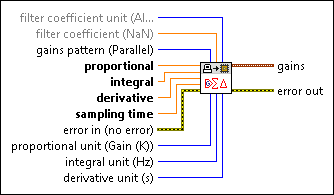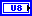filter coefficient unit specifies the unit that distinguishes the type of the derivative lowpass filter coefficients.

 0 Alpha (default)—Specifies that the filter coefficients are expressed in Alpha. 1 N—Specifies that the filter coefficients are expressed in N. 2 Cutoff Frequency—Specifies that the filter coefficients are expressed in hertz. 3 Time Constant—Specifies that the filter coefficients are expressed in seconds.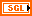filter coefficient specifies the derivative lowpass filter coefficient.

If you specify a value for filter coefficient unit, you must also specify a value for filter coefficient. When filter coefficient unit is Alpha, the valid value range of filter coefficient is [0, 1]. When filter coefficient unit is N, the valid value range of filter coefficient is [1, 1000]. The following equations explain the relationships among the available units:

N = 1 / Alpha

Time Constant = 1 / (2 * Pi * Cutoff Frequency)

When you leave both filter coefficient unit and filter coefficient unwired, this VI uses 0.1 for filter coefficient.gains pattern specifies the form of the PID gains.

 0 Academic—Specifies that the gains are in the Academic form. 1 Parallel (default)—Specifies that the gains are in the Parallel form. 2 Series—Specifies that the gains are in the Series form.proportional specifies the value of the proportional gain.integral specifies the value of the integral gain.derivative specifies the value of the derivative gain.sampling time specifies the loop time, in seconds, at which the PID loop on the FPGA target runs.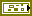error in describes error conditions that occur before this node runs. This input provides standard error in functionality.proportional unit specifies the unit of the proportional gain. The relationship between the available units is K = 100 / PB.

 0 Gain (K) (default)—Specifies that the proportional gain is expressed in terms of proportional gain (K). 1 Band (PB)—Specifies that the proportional gain is expressed in terms of proportional band (PB).integral unit specifies the units of the integral gain.

 0 Hz (default)—Specifies that the integral gain is expressed in hertz. 1 s—Specifies that the integral gain is expressed in seconds. 2 min—Specifies that the integral gain is expressed in minutes.derivative unit specifies the units of the derivative gain.

 0 Hz—Specifies that the derivative gain is expressed in hertz. 1 s (default)—Specifies that the derivative gain is expressed in seconds. 2 min—Specifies that the derivative gain is expressed in minutes.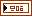gains returns the normalized PID gain parameters.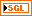proportional gain returns the normalized proportional gain. In equations that define the PID gains conversion, Kp represents proportional gain.integral gain returns the normalized integral gain. In equations that define the PID gains conversion, Ki represents integral gain.derivative gain returns the normalized derivative gain. In equations that define the PID gains conversion, Kd represents derivative gain.filter coefficient returns the derivative lowpass filter coefficient. In equations that define the PID controller, a represents filter coefficient.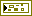error out contains error information. This output provides standard error out functionality.

## Convert PID Gains (PI)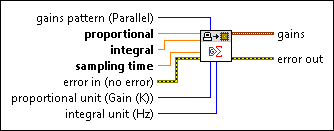gains pattern specifies the form of the PI gains.

 0 Academic—Specifies that the gains are in the Academic form. 1 Parallel (default)—Specifies that the gains are in the Parallel form. 2 Series—Specifies that the gains are in the Series form.proportional specifies the value of the proportional gain.integral specifies the value of the integral gain.sampling time specifies the loop time, in seconds, at which the PID loop on the FPGA target runs.error in describes error conditions that occur before this node runs. This input provides standard error in functionality.proportional unit specifies the unit of the proportional gain. The relationship between the available units is K = 100 / PB.

 0 Gain (K) (default)—Specifies that the proportional gain is expressed in terms of proportional gain (K). 1 Band (PB)—Specifies that the proportional gain is expressed in terms of proportional band (PB).integral unit specifies the units of the integral gain.

 0 Hz (default)—Specifies that the integral gain is expressed in hertz. 1 s—Specifies that the integral gain is expressed in seconds. 2 min—Specifies that the integral gain is expressed in minutes.gains returns the normalized PI gain parameters.proportional gain returns the normalized proportional gain. In equations that define the PID gains conversion, Kp represents proportional gain.integral gain returns the normalized integral gain. In equations that define the PID gains conversion, Ki represents integral gain.error out contains error information. This output provides standard error out functionality.

## Convert PID Gains (PD)filter coefficient unit specifies the unit that distinguishes the type of the derivative lowpass filter coefficients.

 0 Alpha (default)—Specifies that the filter coefficients are expressed in Alpha. 1 N—Specifies that the filter coefficients are expressed in N. 2 Cutoff Frequency—Specifies that the filter coefficients are expressed in hertz. 3 Time Constant—Specifies that the filter coefficients are expressed in seconds.filter coefficient specifies the derivative lowpass filter coefficient.

If you specify a value for filter coefficient unit, you must also specify a value for filter coefficient. When filter coefficient unit is Alpha, the valid value range of filter coefficient is [0, 1]. When filter coefficient unit is N, the valid value range of filter coefficient is [1, 1000]. The following equations explain the relationships among the available units:

N = 1 / Alpha

Time Constant = 1 / (2 * Pi * Cutoff Frequency)

When you leave both filter coefficient unit and filter coefficient unwired, this VI uses 0.1 for filter coefficient.gains pattern specifies the form of the PD gains.

 0 Academic—Specifies that the gains are in the Academic form. 1 Parallel (default)—Specifies that the gains are in the Parallel form. 2 Series—Specifies that the gains are in the Series form.proportional specifies the value of the proportional gain.derivative specifies the value of the derivative gain.sampling time specifies the loop time, in seconds, at which the PID loop on the FPGA target runs.error in describes error conditions that occur before this node runs. This input provides standard error in functionality.proportional unit specifies the unit of the proportional gain. The relationship between the available units is K = 100 / PB.

 0 Gain (K) (default)—Specifies that the proportional gain is expressed in terms of proportional gain (K). 1 Band (PB)—Specifies that the proportional gain is expressed in terms of proportional band (PB).derivative unit specifies the units of the derivative gain.

 0 Hz—Specifies that the derivative gain is expressed in hertz. 1 s (default)—Specifies that the derivative gain is expressed in seconds. 2 min—Specifies that the derivative gain is expressed in minutes.gains returns the normalized PD gain parameters.proportional gain returns the normalized proportional gain. In equations that define the PID gains conversion, Kp represents proportional gain.derivative gain returns the normalized derivative gain. In equations that define the PID gains conversion, Kd represents derivative gain.filter coefficient returns the derivative lowpass filter coefficient. In equations that define the PID controller, a represents filter coefficient.error out contains error information. This output provides standard error out functionality.

## Convert PID Gains Details

Use the following table to understand the details about the equations that the Convert PID Gains VI uses when converting the PID gains. The converted PID gains are Kp, Ki, and Kd, respectively.

Original PID Form Original Gains: Units Conversion Equations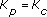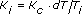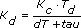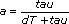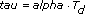Ti: s
Td: s
Parallel Kp': Gain (K)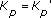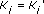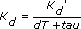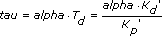Ki': Hz
Kd': s
Series KC': Gain (K)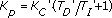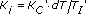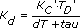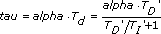TI': s
TD': s

## Examples

Refer to the following VIs for examples of using the Convert PID Gains VI:

• labview\examples\CompactRIO\FPGA Fundamentals\FPGA Math and Analysis\Floating-point PID\Multi-Channel PID\Multi-Channel PID.lvproj
• labview\examples\CompactRIO\FPGA Fundamentals\FPGA Math and Analysis\Floating-point PID\Multi-Rate PID\Multi-Rate PID.lvproj
• labview\examples\R Series\FPGA Fundamentals\FPGA Math and Analysis\Floating-point PID\Multi-Channel PID\Multi-Channel PID.lvproj
• labview\examples\R Series\FPGA Fundamentals\FPGA Math and Analysis\Floating-point PID\Multi-Rate PID\Multi-Rate PID.lvproj